# Volume inequalities for ${L}_{0}$-Minkowski combination of convex bodies

## Abstract

Recently Böröczky, Lutwak, Yang and Zhang proved the ${L}_{0}$-Brunn-Minkowski inequality for two origin-symmetric convex bodies in the plane. This paper extends their results to m ($m\ge 2$) origin-symmetric convex bodies in the plane. Moreover, relying on the recent results of Schuster and Weberndorfer, volume inequalities for ${L}_{0}$-Minkowski combination of origin-symmetric convex bodies in ${\mathbb{R}}^{n}$ and its dual form are established in this paper.

MSC:52A20, 52A40.

## 1 Introduction

The setting for this article is an Euclidean space ${\mathbb{R}}^{n}$, $n\ge 2$. A convex body is a compact convex subset of ${\mathbb{R}}^{n}$ with a non-empty interior. For a compact convex set $K\subset {\mathbb{R}}^{n}$ and $x\in {\mathbb{R}}^{n}$, the support function ${h}_{K}:{\mathbb{R}}^{n}\to \mathbb{R}$ is defined by ${h}_{K}\left(x\right)=max\left\{x\cdot y:y\in K\right\}$, where $x\cdot y$ denotes the standard inner product of x and y in ${\mathbb{R}}^{n}$. The polar body of a convex body K is given by . The Minkowski addition of two convex bodies K and L is defined as $K+L=\left\{x+y:x\in K,y\in L\right\}$, and the scalar multiplication λK of K, where $\lambda \ge 0$, is defined as $\lambda K=\left\{\lambda x:x\in K\right\}$.

In the early 1960s, Firey  extended the Minkowski combination of convex bodies to ${L}_{p}$-Minkowski combination for each $p\ge 1$. Furthermore, he established the ${L}_{p}$-Brunn-Minkowski inequality which states the following: If ${K}_{i}$ ($i=1,2,\dots ,m$) are convex bodies in ${\mathbb{R}}^{n}$ that contain the origin in their interiors, and ${\lambda }_{i}\in \left[0,1\right]$ satisfying ${\sum }_{i=1}^{m}{\lambda }_{i}=1$, then the volumes of the bodies ${K}_{i}$ and their ${L}_{p}$-Minkowski combination ${\lambda }_{1}\cdot {K}_{1}{+}_{p}\cdots {+}_{p}{\lambda }_{m}\cdot {K}_{m}$ are related by

$V\left({\lambda }_{1}\cdot {K}_{1}{+}_{p}\cdots {+}_{p}{\lambda }_{m}\cdot {K}_{m}\right)\ge V{\left({K}_{1}\right)}^{{\lambda }_{1}}\cdots V{\left({K}_{m}\right)}^{{\lambda }_{m}},$
(1.1)

with equality if and only if ${K}_{i}$ are equal.

Recently, Böröczky et al.  defined the ${L}_{0}$-Minkowski combination of convex bodies and proved the ${L}_{0}$-Brunn-Minkowski inequality, which is stronger than (1.1), for two origin-symmetric convex bodies in the plane.

Theorem 1.1 

If K and L are origin-symmetric convex bodies in the plane, then for all real $\lambda \in \left[0,1\right]$,

$V\left(\left(1-\lambda \right)\cdot K{+}_{0}\lambda \cdot L\right)\ge V{\left(K\right)}^{1-\lambda }V{\left(L\right)}^{\lambda }.$

When $\lambda \in \left(0,1\right)$, equality in the inequality holds if and only if K and L are dilates or K and L are parallelograms with parallel sides.

Our first main result of this paper is to extend Theorem 1.1 to m ($m\ge 2$) origin-symmetric convex bodies in the plane.

Definition If ${K}_{i}$ ($i=1,2,\dots ,m$) are convex bodies that contain the origin in their interiors, then for real ${\lambda }_{i}\ge 0$ (not all zero), the ${L}_{0}$-Minkowski combination ${\lambda }_{1}\cdot {K}_{1}{+}_{0}\cdots {+}_{0}{\lambda }_{m}\cdot {K}_{m}$ of ${K}_{i}$ is defined by

${\lambda }_{1}\cdot {K}_{1}{+}_{0}\cdots {+}_{0}{\lambda }_{m}\cdot {K}_{m}=\bigcap _{u\in {S}^{n-1}}\left\{x\in {\mathbb{R}}^{n}:x\cdot u\le \prod _{i=1}^{m}{h}_{{K}_{i}}{\left(u\right)}^{{\lambda }_{i}}\right\}.$
(1.2)

Theorem 1.2 If ${K}_{i}$ ($i=1,2,\dots ,m$) are origin-symmetric convex bodies in the plane, then for all real ${\lambda }_{i}\in \left[0,1\right]$ satisfying ${\sum }_{i=1}^{m}{\lambda }_{i}=1$, we have

$V\left({\lambda }_{1}\cdot {K}_{1}{+}_{0}\cdots {+}_{0}{\lambda }_{m}\cdot {K}_{m}\right)\ge V{\left({K}_{1}\right)}^{{\lambda }_{1}}\cdots V{\left({K}_{m}\right)}^{{\lambda }_{m}}.$
(1.3)

However, the ${L}_{0}$-Brunn-Minkowski inequality in ${\mathbb{R}}^{n}$ is still an open problem, even for origin-symmetric convex bodies.

Now, with our second main result we focus on the volume estimate for ${L}_{0}$-Minkowski combination of origin-symmetric convex bodies in ${\mathbb{R}}^{n}$.

In , Schuster and Weberndorfer established two powerful volume inequalities of the Wulff shape ${W}_{\nu ,f}$ determined by an f-centered isotropic measure ν (see Section 2 for details). Using their results, we establish the following two inequalities.

Theorem 1.3 If ${K}_{i}$ ($i=1,2,\dots ,m$) are origin-symmetric convex bodies in ${\mathbb{R}}^{n}$, then for all real ${\lambda }_{i}\in \left[0,1\right]$ satisfying ${\sum }_{i=1}^{m}{\lambda }_{i}=1$, we have

$V\left({\lambda }_{1}\cdot {K}_{1}{+}_{0}\cdots {+}_{0}{\lambda }_{m}\cdot {K}_{m}\right)\le \frac{{n}^{n/2}{\left(n+1\right)}^{\left(n+1\right)/2}}{n!}\prod _{i=1}^{m}{\left(\underset{u\in {S}^{n-1}}{sup}{h}_{{K}_{i}}\left(u\right)\right)}^{n{\lambda }_{i}}.$

Theorem 1.4 If ${K}_{i}$ ($i=1,2,\dots ,m$) are origin-symmetric convex bodies in ${\mathbb{R}}^{n}$, then for all real ${\lambda }_{i}\in \left[0,1\right]$ satisfying ${\sum }_{i=1}^{m}{\lambda }_{i}=1$, we have

$V\left({\left({\lambda }_{1}\cdot {K}_{1}{+}_{0}\cdots {+}_{0}{\lambda }_{m}\cdot {K}_{m}\right)}^{\ast }\right)\ge \frac{{\left(n+1\right)}^{\left(n+1\right)/2}}{n!{n}^{n/2}}\prod _{i=1}^{m}\underset{u\in {S}^{n-1}}{inf}\left({h}_{{K}_{i}}{\left(u\right)}^{-n{\lambda }_{i}}\right).$

Furthermore, inequalities of mixed volume and normalized ${L}_{0}$-mixed volume (given in this paper) for ${L}_{0}$-Minkowski combination of not necessarily origin-symmetric convex bodies in ${\mathbb{R}}^{n}$ are established in the following theorems.

Theorem 1.5 If ${K}_{i}$ ($i=1,2,\dots ,m$) and ${L}_{j}$ ($j=2,\dots ,n$) are convex bodies in ${\mathbb{R}}^{n}$ that contain the origin in their interiors, then for all real ${\lambda }_{i}\in \left[0,1\right]$ satisfying ${\sum }_{i=1}^{m}{\lambda }_{i}=1$, we have

$V\left({\lambda }_{1}\cdot {K}_{1}{+}_{0}\cdots {+}_{0}{\lambda }_{m}\cdot {K}_{m},{L}_{2},\dots ,{L}_{n}\right)\le \prod _{i=1}^{m}V{\left({K}_{i},{L}_{2},\dots ,{L}_{n}\right)}^{{\lambda }_{i}}.$

If K and L are convex bodies in ${\mathbb{R}}^{n}$ that contain the origin in their interiors, then for $p\ne 0$, the ${L}_{p}$-mixed volume ${V}_{p}\left(K,L\right)$ can be defined as

${V}_{p}\left(K,L\right)=\frac{1}{n}{\int }_{{S}^{n-1}}{h}_{L}^{p}{h}_{K}^{1-p}\phantom{\rule{0.2em}{0ex}}d{S}_{K}={\int }_{{S}^{n-1}}{\left(\frac{{h}_{L}}{{h}_{K}}\right)}^{p}\phantom{\rule{0.2em}{0ex}}d{V}_{K},$

where ${S}_{K}$ and ${V}_{K}$ are the surface area measure and the cone-volume measure of K, respectively (see Section 2 for the definitions).

The normalized ${L}_{p}$-mixed volume ${\overline{V}}_{p}\left(K,L\right)$ is defined by

${\overline{V}}_{p}\left(K,L\right)={\left(\frac{{V}_{p}\left(K,L\right)}{V\left(K\right)}\right)}^{1/p}={\left({\int }_{{S}^{n-1}}{\left(\frac{{h}_{L}}{{h}_{K}}\right)}^{p}\phantom{\rule{0.2em}{0ex}}d{\overline{V}}_{K}\right)}^{1/p},$

where ${\overline{V}}_{K}$ is the cone-volume probability measure of K (also see Section 2 for the definition).

Note that when p converges to zero, the normalized ${L}_{0}$-mixed volume ${\overline{V}}_{0}\left(K,L\right)$ can naturally be given as

${\overline{V}}_{0}\left(K,L\right)=exp\left({\int }_{{S}^{n-1}}log\frac{{h}_{L}}{{h}_{K}}\phantom{\rule{0.2em}{0ex}}d{\overline{V}}_{K}\right).$
(1.4)

Theorem 1.6 Suppose that K, L and Q are convex bodies in ${\mathbb{R}}^{n}$ that contain the origin in their interiors, then for real $\lambda \in \left[0,1\right]$, we have

${\overline{V}}_{0}\left(Q,\left(1-\lambda \right)\cdot K{+}_{0}\lambda \cdot L\right)\le {\overline{V}}_{0}{\left(Q,K\right)}^{1-\lambda }{\overline{V}}_{0}{\left(Q,L\right)}^{\lambda }.$

Combining the famous variant (proved in ) of Aleksandrov’s lemma and the representation of (1.4), we obtain a limit form of ${\overline{V}}_{0}\left(K,L\right)$ in the following theorem.

Theorem 1.7 Suppose that K and L are convex bodies in ${\mathbb{R}}^{n}$ that contain the origin in their interiors, then we have

$nV\left(K\right)log{\overline{V}}_{0}\left(K,L\right)=\underset{\lambda \to 0}{lim}\frac{V\left(\left(1-\lambda \right)\cdot K{+}_{0}\lambda \cdot L\right)-V\left(K\right)}{\lambda }.$

The paper is organized as follows. In Section 2 some of the basic notations and preliminaries are provided. Section 3 contains the proofs of the main theorems. Some properties of normalized ${L}_{0}$-mixed volume and Wulff shape are discussed in Section 4.

## 2 Notations and preliminaries

Good general references for the theory of convex bodies are provided by the books  and the articles [2, 4, 7, 1025].

The group of nonsingular linear transformations is denoted by $\mathit{GL}\left(n\right)$; its members are, in particular, bijections of ${\mathbb{R}}^{n}$ onto itself. The group of special linear transformations of ${\mathbb{R}}^{n}$ is denoted by $\mathit{SL}\left(n\right)$. These are the members of $\mathit{GL}\left(n\right)$ whose determinant is one.

For $\varphi \in \mathit{GL}\left(n\right)$, let ${\varphi }^{t}$, ${\varphi }^{-1}$ and ${\varphi }^{-t}$ denote the transpose, inverse and inverse of the transpose of ϕ, respectively.

For $x\in {\mathbb{R}}^{n}$, then

and

Recall that for a Borel set $\omega \subseteq {S}^{n-1}$, the surface area measure of a convex body K in ${\mathbb{R}}^{n}$ ${S}_{K}\left(\omega \right)$ is the $\left(n-1\right)$-dimensional Hausdorff measure of the set of all boundary points of K at which there exists a normal vector of K belonging to ω.

Let K be a convex body in ${\mathbb{R}}^{n}$ that contains the origin in its interior. The cone-volume measure ${V}_{K}$ of K is a Borel measure on the unit sphere ${S}^{n-1}$ defined by

$d{V}_{K}=\frac{1}{n}{h}_{K}\phantom{\rule{0.2em}{0ex}}d{S}_{K}.$
(2.1)

Obviously,

$V\left(K\right)=\frac{1}{n}{\int }_{{S}^{n-1}}{h}_{K}\left(u\right)\phantom{\rule{0.2em}{0ex}}d{S}_{K}\left(u\right).$

The cone-volume probability measure ${\overline{V}}_{K}$ of K is defined by

${\overline{V}}_{K}=\frac{1}{V\left(K\right)}{V}_{K}.$
(2.2)

Let ${S}^{n-1}$ and B denote the unit sphere centered at the origin and the unit ball in ${\mathbb{R}}^{n}$, respectively. The n-dimensional volume ${\kappa }_{n}$ of B and the $\left(n-1\right)$-dimensional volume ${\omega }_{n}$ of ${S}^{n-1}$ are

${\kappa }_{n}=\frac{{\pi }^{\frac{n}{2}}}{\mathrm{\Gamma }\left(1+\frac{n}{2}\right)},$

and

${\omega }_{n}=n{\kappa }_{n}.$

If ${K}_{i}$ ($i=1,2,\dots ,n$) are convex bodies in ${\mathbb{R}}^{n}$, the mixed volume $V\left({K}_{1},\dots ,{K}_{n}\right)$ is given by (see , [, Theorem 5.1.6] or [, Section 29])

$V\left({K}_{1},\dots ,{K}_{n}\right)=\frac{1}{n}{\int }_{{S}^{n-1}}{h}_{{K}_{1}}\left(u\right)\phantom{\rule{0.2em}{0ex}}dS\left({K}_{2},\dots ,{K}_{n},u\right),$
(2.3)

where $S\left({K}_{2},\dots ,{K}_{n},\cdot \right)$ is the mixed area measure of ${K}_{i}$ ($i=2,\dots ,n$).

If K is a convex body in ${\mathbb{R}}^{n}$, the quermassintegrals ${W}_{i}\left(K\right)$ of K are defined for $0\le i\le n$ by

${W}_{i}\left(K\right)=V\left(K,n-i;B,i\right),$

where the notation $V\left(K,n-i;B,i\right)$ signifies that K appears $\left(n-i\right)$ times and B appears i times.

The mean width $W\left(K\right)$ of a convex body K in ${\mathbb{R}}^{n}$ is defined by

$W\left(K\right)=\frac{2}{{\omega }_{n}}{\int }_{{S}^{n-1}}{h}_{K}\left(u\right)\phantom{\rule{0.2em}{0ex}}d{\mathcal{H}}^{n-1}\left(u\right),$

where ${\mathcal{H}}^{n-1}$ denotes the $\left(n-1\right)$-dimensional Hausdorff measure.

It can be shown that

$\frac{2}{{\kappa }_{n}}{W}_{n-1}\left(K\right)=W\left(K\right).$
(2.4)

Throughout, all Borel measures are understood to be non-negative and finite. We write suppν for the support of a measure ν.

Suppose that ν is a Borel measure on ${S}^{n-1}$ and f is a positive continuous function on ${S}^{n-1}$. The Wulff shape ${W}_{\nu ,f}$ determined by ν and f is defined by

(2.5)

Obviously,

(2.6)

Let f be a positive continuous function on ${S}^{n-1}$. A Borel measure ν on ${S}^{n-1}$ is called f-centered if

${\int }_{{S}^{n-1}}f\left(u\right)u\phantom{\rule{0.2em}{0ex}}d\nu \left(u\right)=o.$

The measure ν is called isotropic if

${\int }_{{S}^{n-1}}u\otimes u\phantom{\rule{0.2em}{0ex}}d\nu \left(u\right)={I}_{n},$

where $u\otimes u$ is the orthogonal projection onto the line spanned by u and ${I}_{n}$ denotes the identity map on ${\mathbb{R}}^{n}$. Thus, ν is isotropic if

The displacement of ${W}_{\nu ,f}$ is defined by

$disp{W}_{\nu ,f}=cd{W}_{\nu ,f}\cdot {\int }_{{S}^{n-1}}\frac{u}{f\left(u\right)}\phantom{\rule{0.2em}{0ex}}d\nu \left(u\right),$

where $cd{W}_{\nu ,f}$ denotes the centroid of ${W}_{\nu ,f}$.

## 3 Proof of main results

The following lemma will be used in the proof of Theorem 1.2.

Lemma 3.1 If ${K}_{i}$ ($i=1,2,\dots ,m$) are convex bodies that contain the origin in their interiors, then for real ${\lambda }_{i}\in \left(0,1\right)$ and ${\sum }_{i=1}^{m}{\lambda }_{i}=1$, we have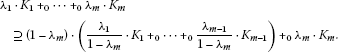Proof Since ${K}_{i}$ ($i=1,2,\dots ,m$) contain the origin in their interiors, thus it is easy to see that the ${L}_{0}$-Minkowski combination

$\frac{{\lambda }_{1}}{1-{\lambda }_{m}}\cdot {K}_{1}{+}_{0}\cdots {+}_{0}\frac{{\lambda }_{m-1}}{1-{\lambda }_{m}}\cdot {K}_{m-1}=\bigcap _{u\in {S}^{n-1}}\left\{x\in {\mathbb{R}}^{n}:x\cdot u\le \prod _{i=1}^{m-1}{h}_{{K}_{i}}{\left(u\right)}^{\frac{{\lambda }_{i}}{1-{\lambda }_{m}}}\right\},$
(3.1)

also contains the origin in its interior.

Let $x\in \left(1-{\lambda }_{m}\right)\cdot \left(\frac{{\lambda }_{1}}{1-{\lambda }_{m}}\cdot {K}_{1}{+}_{0}\cdots {+}_{0}\frac{{\lambda }_{m-1}}{1-{\lambda }_{m}}\cdot {K}_{m-1}\right){+}_{0}{\lambda }_{m}\cdot {K}_{m}$, then combining (2.6) and (3.1) we have

$\begin{array}{rcl}x\cdot u& \le & {h}_{\frac{{\lambda }_{1}}{1-{\lambda }_{m}}\cdot {K}_{1}{+}_{0}\cdots {+}_{0}\frac{{\lambda }_{m-1}}{1-{\lambda }_{m}}\cdot {K}_{m-1}}{\left(u\right)}^{1-{\lambda }_{m}}{h}_{{K}_{m}}{\left(u\right)}^{{\lambda }_{m}}\\ \le & {\left(\prod _{i=1}^{m-1}{h}_{{K}_{i}}{\left(u\right)}^{\frac{{\lambda }_{i}}{1-{\lambda }_{m}}}\right)}^{1-{\lambda }_{m}}{h}_{{K}_{m}}{\left(u\right)}^{{\lambda }_{m}}\\ =& \prod _{i=1}^{m}{h}_{{K}_{i}}{\left(u\right)}^{{\lambda }_{i}}\end{array}$

for all $u\in {S}^{n-1}$.

Hence, by (1.2), we have $x\in {\lambda }_{1}\cdot {K}_{1}{+}_{0}\cdots {+}_{0}{\lambda }_{m}\cdot {K}_{m}$, which yields the lemma directly. □

Proof of Theorem 1.2 We will prove Theorem 1.2 by induction on m.

Obviously, it is true by Theorem 1.1 when $m=2$.

Suppose that the result holds on $\left(m-1\right)$. Thus, for real ${\lambda }_{i}\in \left(0,1\right)$ satisfying ${\sum }_{i=1}^{m}{\lambda }_{i}=1$, we have

$V\left(\frac{{\lambda }_{1}}{1-{\lambda }_{m}}\cdot {K}_{1}{+}_{0}\cdots {+}_{0}\frac{{\lambda }_{m-1}}{1-{\lambda }_{m}}\cdot {K}_{m-1}\right)\ge V{\left({K}_{1}\right)}^{\frac{{\lambda }_{1}}{1-{\lambda }_{m}}}\cdots V{\left({K}_{m-1}\right)}^{\frac{{\lambda }_{m-1}}{1-{\lambda }_{m}}}.$

We now consider the situation on m. In fact, since ${K}_{i}$ ($i=1,2,\dots ,m$) are origin-symmetric convex bodies in the plane, then by (3.1) we have $\frac{{\lambda }_{1}}{1-{\lambda }_{m}}\cdot {K}_{1}{+}_{0}\cdots {+}_{0}\frac{{\lambda }_{m-1}}{1-{\lambda }_{m}}\cdot {K}_{m-1}$ is also an origin-symmetric convex body in the plane.

Then by Lemma 3.1, Theorem 1.1 and the induction hypothesis, we have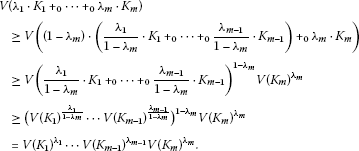Note that Theorem 1.2 also holds when ${\lambda }_{m}=0$ and ${\lambda }_{m}=1$, respectively.

Thus Theorem 1.2 holds for all ${\lambda }_{i}\in \left[0,1\right]$ satisfying ${\sum }_{i=1}^{m}{\lambda }_{i}=1$. □

In , Schuster and Weberndorfer established a sharp bound for the volume of the Wulff shape ${W}_{\nu ,f}$ determined by an f-centered isotropic measure ν as follows.

Lemma 3.2 

Suppose that f is a positive continuous function on ${S}^{n-1}$ and that ν is an isotropic f-centered measure. If $disp{W}_{\nu ,f}=0$, then

$V\left({W}_{\nu ,f}\right)\le \frac{{\left(n+1\right)}^{\left(n+1\right)/2}}{n!}{\parallel f\parallel }_{{L}^{2}\left(\nu \right)}^{n},$

with equality if and only if $convsupp\nu$ is a regular simplex inscribed in ${S}^{n-1}$ and f is constant on suppν.

Proof of Theorem 1.3 Let $d\nu =\frac{1}{{\kappa }_{n}}\phantom{\rule{0.2em}{0ex}}du$ and $f\left(u\right)={\prod }_{i=1}^{m}{h}_{{K}_{i}}{\left(u\right)}^{{\lambda }_{i}}$ for all $u\in {S}^{n-1}$ in Lemma 3.2. Obviously, f is a positive continuous function on ${S}^{n-1}$ and ν is isotropic. By (2.5) we have

Combining it with (1.2), we get

${W}_{\nu ,f}={\lambda }_{1}\cdot {K}_{1}{+}_{0}\cdots {+}_{0}{\lambda }_{m}\cdot {K}_{m}.$
(3.2)

Furthermore, since ${K}_{i}$ ($i=1,2,\dots ,m$) are origin-symmetric convex bodies, then we have

$\frac{1}{{\kappa }_{n}}{\int }_{{S}^{n-1}}{h}_{{K}_{1}}{\left(u\right)}^{{\lambda }_{1}}\cdots {h}_{{K}_{m}}{\left(u\right)}^{{\lambda }_{m}}u\phantom{\rule{0.2em}{0ex}}du=o,$

and

$\frac{1}{{\kappa }_{n}}{\int }_{{S}^{n-1}}\frac{u}{{h}_{{K}_{1}}{\left(u\right)}^{{\lambda }_{1}}\cdots {h}_{{K}_{m}}{\left(u\right)}^{{\lambda }_{m}}}\phantom{\rule{0.2em}{0ex}}du=o.$

Thus ν is f-centered, and

$disp{W}_{\nu ,f}=cd{W}_{\nu ,f}\cdot \frac{1}{{\kappa }_{n}}{\int }_{{S}^{n-1}}\frac{u}{{h}_{{K}_{1}}{\left(u\right)}^{{\lambda }_{1}}\cdots {h}_{{K}_{m}}{\left(u\right)}^{{\lambda }_{m}}}\phantom{\rule{0.2em}{0ex}}du=0.$

Combining (3.2) and Lemma 3.2, we have

$V\left({\lambda }_{1}\cdot {K}_{1}{+}_{0}\cdots {+}_{0}{\lambda }_{m}\cdot {K}_{m}\right)\le \frac{{\left(n+1\right)}^{\left(n+1\right)/2}}{n!}{\parallel f\parallel }_{{L}^{2}\left(\nu \right)}^{n}.$
(3.3)

Since ${\lambda }_{i}\in \left[0,1\right]$ satisfying ${\sum }_{i=1}^{m}{\lambda }_{i}=1$, then from Hölder’s inequality (see ) we have

$\begin{array}{rcl}{\parallel f\parallel }_{{L}^{2}\left(\nu \right)}^{2}& =& \frac{1}{{\kappa }_{n}}{\int }_{{S}^{n-1}}f{\left(u\right)}^{2}\phantom{\rule{0.2em}{0ex}}du\\ =& \frac{1}{{\kappa }_{n}}{\int }_{{S}^{n-1}}\prod _{i=1}^{m}{h}_{{K}_{i}}{\left(u\right)}^{2{\lambda }_{i}}\phantom{\rule{0.2em}{0ex}}du\\ \le & n\prod _{i=1}^{m}{\left(\underset{u\in {S}^{n-1}}{sup}{h}_{{K}_{i}}\left(u\right)\right)}^{2{\lambda }_{i}}.\end{array}$
(3.4)

Combining (3.3) and (3.4), we obtain

$V\left({\lambda }_{1}\cdot {K}_{1}{+}_{0}\cdots {+}_{0}{\lambda }_{m}\cdot {K}_{m}\right)\le \frac{{n}^{n/2}{\left(n+1\right)}^{\left(n+1\right)/2}}{n!}\prod _{i=1}^{m}{\left(\underset{u\in {S}^{n-1}}{sup}{h}_{{K}_{i}}\left(u\right)\right)}^{n{\lambda }_{i}}.$

□

A natural dual to Lemma 3.2 is also given in , which provided a sharp lower bound for the volume of the polar of the Wulff shape ${W}_{\nu ,f}$.

Lemma 3.3 

Suppose that f is a positive continuous function on ${S}^{n-1}$ and that ν is an isotropic f-centered measure. Then

$V\left({W}_{\nu ,f}^{\ast }\right)\ge \frac{{\left(n+1\right)}^{\left(n+1\right)/2}}{n!}{\parallel f\parallel }_{{L}^{2}\left(\nu \right)}^{-n},$

with equality if and only if $convsupp\nu$ is a regular simplex inscribed in ${S}^{n-1}$ and f is constant on suppν.

Proof of Theorem 1.4 Let $d\nu =\frac{1}{{\kappa }_{n}}\phantom{\rule{0.2em}{0ex}}du$ and $f\left(u\right)={\prod }_{i=1}^{m}{h}_{{K}_{i}}{\left(u\right)}^{{\lambda }_{i}}$ for all $u\in {S}^{n-1}$ in Lemma 3.3. Similarly, from the proof of Theorem 1.3, we know that f is a positive continuous function on ${S}^{n-1}$ and ν is an isotropic f-centered measure.

Thus, combining (3.2) and Lemma 3.3, we have

$V\left({\left({\lambda }_{1}\cdot {K}_{1}{+}_{0}\cdots {+}_{0}{\lambda }_{m}\cdot {K}_{m}\right)}^{\ast }\right)\ge \frac{{\left(n+1\right)}^{\left(n+1\right)/2}}{n!}{\parallel f\parallel }_{{L}^{2}\left(\nu \right)}^{-n}.$
(3.5)

Now, combining (3.4) and (3.5), we obtain

$\begin{array}{rcl}V\left({\left({\lambda }_{1}\cdot {K}_{1}{+}_{0}\cdots {+}_{0}{\lambda }_{m}\cdot {K}_{m}\right)}^{\ast }\right)& \ge & \frac{{\left(n+1\right)}^{\left(n+1\right)/2}}{n!{n}^{n/2}}\prod _{i=1}^{m}{\left(\underset{u\in {S}^{n-1}}{sup}{h}_{{K}_{i}}\left(u\right)\right)}^{-n{\lambda }_{i}}\\ =& \frac{{\left(n+1\right)}^{\left(n+1\right)/2}}{n!{n}^{n/2}}\prod _{i=1}^{m}\underset{u\in {S}^{n-1}}{inf}\left({h}_{{K}_{i}}{\left(u\right)}^{-n{\lambda }_{i}}\right).\end{array}$

□

Proof of Theorem 1.5 Since ${\lambda }_{i}\in \left[0,1\right]$ satisfying ${\sum }_{i=1}^{m}{\lambda }_{i}=1$, then by (2.3), (2.6), Hölder’s inequality and again (2.3), we have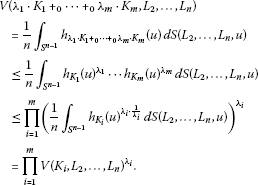□

Letting ${L}_{2}=\cdots ={L}_{n}={\lambda }_{1}\cdot {K}_{1}{+}_{0}\cdots {+}_{0}{\lambda }_{m}\cdot {K}_{m}$ in Theorem 1.5 gives the following inequality of mixed volumes.

Corollary 3.4 If ${K}_{i}$ ($i=1,2,\dots ,m$) are convex bodies in ${\mathbb{R}}^{n}$ that contain the origin in their interiors, then for all real ${\lambda }_{i}\in \left[0,1\right]$ satisfying ${\sum }_{i=1}^{m}{\lambda }_{i}=1$, we have

$V\left({\lambda }_{1}\cdot {K}_{1}{+}_{0}\cdots {+}_{0}{\lambda }_{m}\cdot {K}_{m}\right)\le \prod _{{i}_{1}=1}^{m}\cdots \prod _{{i}_{n}=1}^{m}V{\left({K}_{{i}_{1}},\dots ,{K}_{{i}_{n}}\right)}^{{\lambda }_{{i}_{1}}\cdots {\lambda }_{{i}_{n}}}.$

Letting ${L}_{2}=\cdots ={L}_{n}=B$ in Theorem 1.5 gives the following inequality of quermassintegrals.

Corollary 3.5 If ${K}_{i}$ ($i=1,2,\dots ,m$) are convex bodies in ${\mathbb{R}}^{n}$ that contain the origin in their interiors, then for all real ${\lambda }_{i}\in \left[0,1\right]$ satisfying ${\sum }_{i=1}^{m}{\lambda }_{i}=1$, we have

${W}_{n-1}\left({\lambda }_{1}\cdot {K}_{1}{+}_{0}\cdots {+}_{0}{\lambda }_{m}\cdot {K}_{m}\right)\le \prod _{i=1}^{m}{W}_{n-1}{\left({K}_{i}\right)}^{{\lambda }_{i}}.$

In view of (2.4), we also obtain the following inequality of mean widths.

Corollary 3.6 If ${K}_{i}$ ($i=1,2,\dots ,m$) are convex bodies in ${\mathbb{R}}^{n}$ that contain the origin in their interiors, then for all real ${\lambda }_{i}\in \left[0,1\right]$ satisfying ${\sum }_{i=1}^{m}{\lambda }_{i}=1$, we have

$W\left({\lambda }_{1}\cdot {K}_{1}{+}_{0}\cdots {+}_{0}{\lambda }_{m}\cdot {K}_{m}\right)\le \prod _{i=1}^{m}W{\left({K}_{i}\right)}^{{\lambda }_{i}}.$

Proof of Theorem 1.6 Since K, L and Q are convex bodies in ${\mathbb{R}}^{n}$ that contain the origin in their interiors, then from (1.4), (1.2), (2.6) and again (1.4), we have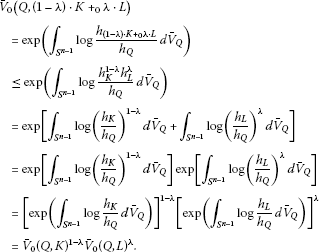□

The following variant of Aleksandrov’s lemma (see [, p.103]) will be needed in proving Theorem 1.7.

Lemma 3.7 

Suppose that ${q}_{\lambda }\left(u\right)=q\left(\lambda ,u\right):I×{S}^{n-1}\to \left(0,\mathrm{\infty }\right)$ is a continuous function, where $I\subset \mathbb{R}$ is an open interval. Suppose also that the convergence in

$\frac{\partial q\left(\lambda ,u\right)}{\partial \lambda }=\underset{\gamma \to 0}{lim}\frac{q\left(\lambda +\gamma ,u\right)-q\left(\lambda ,u\right)}{\gamma }$

is uniform on ${S}^{n-1}$. If ${\left\{{Q}_{\lambda }\right\}}_{\lambda \in I}$ is the family of Wulff shapes associated with ${q}_{\lambda }$, i.e., for fixed $\lambda \in I$,

${Q}_{\lambda }=\bigcap _{u\in {S}^{n-1}}\left\{x\in {\mathbb{R}}^{n}:x\cdot u\le q\left(\lambda ,u\right)\right\},$

then

$\frac{dV\left({Q}_{\lambda }\right)}{d\lambda }={\int }_{{S}^{n-1}}\frac{\partial q\left(\lambda ,u\right)}{\partial \lambda }\phantom{\rule{0.2em}{0ex}}d{S}_{{Q}_{\lambda }}\left(u\right).$

Proof of Theorem 1.7 Since K and L are convex bodies in ${\mathbb{R}}^{n}$ that contain the origin in their interiors, let $q\left(\lambda ,u\right)={h}_{K}{\left(u\right)}^{1-\lambda }{h}_{L}{\left(u\right)}^{\lambda }$ in Lemma 3.7, then the convergence in

$\begin{array}{rcl}\frac{\partial \left({h}_{K}{\left(u\right)}^{1-\lambda }{h}_{L}{\left(u\right)}^{\lambda }\right)}{\partial \lambda }& =& \underset{\gamma \to 0}{lim}\frac{{h}_{K}{\left(u\right)}^{1-\left(\lambda +\gamma \right)}{h}_{L}{\left(u\right)}^{\left(\lambda +\gamma \right)}-{h}_{K}{\left(u\right)}^{1-\lambda }{h}_{L}{\left(u\right)}^{\lambda }}{\gamma }\\ =& {h}_{K}\left(u\right){\left(\frac{{h}_{L}\left(u\right)}{{h}_{K}\left(u\right)}\right)}^{\lambda }log\frac{{h}_{L}\left(u\right)}{{h}_{K}\left(u\right)}\end{array}$

is uniform on ${S}^{n-1}$, and

${Q}_{\lambda }=\bigcap _{u\in {S}^{n-1}}\left\{x\in {\mathbb{R}}^{n}:x\cdot u\le {h}_{K}{\left(u\right)}^{1-\lambda }{h}_{L}{\left(u\right)}^{\lambda }\right\}.$
(3.6)

Observe that $q\left(0,u\right)$ is the support function of K, hence ${Q}_{0}=K$.

On the one hand, from Lemma 3.7, (2.1), (2.2) and (1.4) we have

$\begin{array}{rcl}{\frac{dV\left({Q}_{\lambda }\right)}{d\lambda }|}_{\lambda =0}& =& {\int }_{{S}^{n-1}}{\frac{\partial \left({h}_{K}{\left(u\right)}^{1-\lambda }{h}_{L}{\left(u\right)}^{\lambda }\right)}{\partial \lambda }|}_{\lambda =0}\phantom{\rule{0.2em}{0ex}}d{S}_{{Q}_{0}}\left(u\right)\\ =& {\int }_{{S}^{n-1}}{h}_{K}\left(u\right)log\frac{{h}_{L}\left(u\right)}{{h}_{K}\left(u\right)}\phantom{\rule{0.2em}{0ex}}d{S}_{K}\left(u\right)\\ =& nV\left(K\right){\int }_{{S}^{n-1}}log\frac{{h}_{L}\left(u\right)}{{h}_{K}\left(u\right)}\phantom{\rule{0.2em}{0ex}}d{\overline{V}}_{K}\left(u\right)\\ =& nV\left(K\right)log{\overline{V}}_{0}\left(K,L\right).\end{array}$
(3.7)

On the other hand, by (3.6) and (1.2), we have

${Q}_{\lambda }=\left(1-\lambda \right)\cdot K{+}_{0}\lambda \cdot L.$

Therefore

${\frac{dV\left({Q}_{\lambda }\right)}{d\lambda }|}_{\lambda =0}=\underset{\lambda \to 0}{lim}\frac{V\left(\left(1-\lambda \right)\cdot K{+}_{0}\lambda \cdot L\right)-V\left(K\right)}{\lambda }.$
(3.8)

Hence, combining (3.7) and (3.8), we obtain

$nV\left(K\right)log{\overline{V}}_{0}\left(K,L\right)=\underset{\lambda \to 0}{lim}\frac{V\left(\left(1-\lambda \right)\cdot K{+}_{0}\lambda \cdot L\right)-V\left(K\right)}{\lambda }.$

□

## 4 Other results and comments

Firstly, we prove that ${L}_{0}$-Minkowski combination and normalized ${L}_{0}$-mixed volume are invariant under simultaneous unimodular centro-affine transformations.

Proposition 4.1 Suppose that K and L are convex bodies in ${\mathbb{R}}^{n}$ that contain the origin in their interiors, and $\lambda \in \left[0,1\right]$. If $\varphi \in \mathit{SL}\left(n\right)$, then

$\varphi \left(\left(1-\lambda \right)\cdot K{+}_{0}\lambda \cdot L\right)=\left(1-\lambda \right)\cdot \varphi K{+}_{0}\lambda \cdot \varphi L.$

Proof For $x\in {\mathbb{R}}^{n}$ and $u\in {S}^{n-1}$, let $y=\varphi x$ and ${\varphi }^{-t}u=|{\varphi }^{-t}u|v$, then $y\in {\mathbb{R}}^{n}$ and $v\in {S}^{n-1}$. Thus we have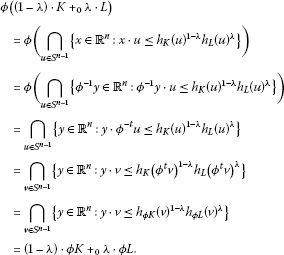□

Proposition 4.2 Suppose that K and L are convex bodies in ${\mathbb{R}}^{n}$ that contain the origin in their interiors, then for $\varphi \in \mathit{SL}\left(n\right)$ we have

${\overline{V}}_{0}\left(\varphi K,\varphi L\right)={\overline{V}}_{0}\left(K,L\right).$

Proof

From Theorem 1.7, we have

${\overline{V}}_{0}\left(K,L\right)=exp\left\{\frac{1}{nV\left(K\right)}\underset{\lambda \to 0}{lim}\frac{V\left(\left(1-\lambda \right)\cdot K{+}_{0}\lambda \cdot L\right)-V\left(K\right)}{\lambda }\right\}.$

Then, by Proposition 4.1, we obtain

$\begin{array}{rcl}{\overline{V}}_{0}\left(\varphi K,\varphi L\right)& =& exp\left\{\frac{1}{nV\left(\varphi K\right)}\underset{\lambda \to 0}{lim}\frac{V\left(\left(1-\lambda \right)\cdot \varphi K{+}_{0}\lambda \cdot \varphi L\right)-V\left(\varphi K\right)}{\lambda }\right\}\\ =& exp\left\{\frac{1}{nV\left(\varphi K\right)}\underset{\lambda \to 0}{lim}\frac{V\left(\varphi \left(\left(1-\lambda \right)\cdot K{+}_{0}\lambda \cdot L\right)\right)-V\left(\varphi K\right)}{\lambda }\right\}\\ =& exp\left\{\frac{1}{nV\left(K\right)}\underset{\lambda \to 0}{lim}\frac{V\left(\left(1-\lambda \right)\cdot K{+}_{0}\lambda \cdot L\right)-V\left(K\right)}{\lambda }\right\}\\ =& {\overline{V}}_{0}\left(K,L\right).\end{array}$

□

The following proposition shows the property of weak convergence of the cone-volume probability measure.

Proposition 4.3 If ${K}_{i}$ is a sequence of convex bodies in ${\mathbb{R}}^{n}$ that contain the origin in their interiors, and ${lim}_{i\to \mathrm{\infty }}{K}_{i}={K}_{0}$, where ${K}_{0}$ is a convex body that also contains the origin in its interior, then ${lim}_{i\to \mathrm{\infty }}{\overline{V}}_{{K}_{i}}={\overline{V}}_{{K}_{0}}$ weakly.

Proof Suppose that $f\in C\left({S}^{n-1}\right)$. Since ${K}_{i}\to {K}_{0}$, by definition, ${h}_{{K}_{i}}\to {h}_{{K}_{0}}$ uniformly on ${S}^{n-1}$. Since the continuous function ${h}_{{K}_{0}}$ is positive, the ${h}_{{K}_{i}}$ are uniformly bounded away from zero, and thus

But ${K}_{i}\to {K}_{0}$ also implies (see ) that

By the continuity of the volume, that is, if ${K}_{i}\to {K}_{0}$ then $V\left({K}_{i}\right)\to V\left({K}_{0}\right)$, we have

$\frac{1}{nV\left({K}_{i}\right)}{\int }_{{S}^{n-1}}f\left(u\right){h}_{{K}_{i}}\left(u\right)\phantom{\rule{0.2em}{0ex}}dS\left({K}_{i},u\right)\to \frac{1}{nV\left({K}_{0}\right)}{\int }_{{S}^{n-1}}f\left(u\right){h}_{{K}_{0}}\left(u\right)\phantom{\rule{0.2em}{0ex}}dS\left({K}_{0},u\right)$

or, equivalently,

${\int }_{{S}^{n-1}}f\left(u\right)\phantom{\rule{0.2em}{0ex}}d{\overline{V}}_{{K}_{i}}\left(u\right)\to {\int }_{{S}^{n-1}}f\left(u\right)\phantom{\rule{0.2em}{0ex}}d{\overline{V}}_{{K}_{0}}\left(u\right).$

□

The continuity of the normalized ${L}_{0}$-mixed volume is contained in the following proposition.

Proposition 4.4 Suppose that ${K}_{i}$ and ${L}_{i}$ are two sequences of convex bodies in ${\mathbb{R}}^{n}$ that contain the origin in their interiors, and ${lim}_{i\to \mathrm{\infty }}{K}_{i}=K$, ${lim}_{i\to \mathrm{\infty }}{L}_{i}=L$, where K and L are convex bodies that also contain the origin in its interior, then ${lim}_{i\to \mathrm{\infty }}{\overline{V}}_{0}\left({K}_{i},{L}_{i}\right)={\overline{V}}_{0}\left(K,L\right)$.

Proof Since ${K}_{i}\to K$ and ${L}_{i}\to L$, by definition, ${h}_{{K}_{i}}\to {h}_{K}$ and ${h}_{{L}_{i}}\to {h}_{L}$ uniformly on ${S}^{n-1}$. Since the continuous functions ${h}_{K}$ and ${h}_{L}$ are positive, the ${h}_{{K}_{i}}$ and ${h}_{{L}_{i}}$ are uniformly bounded away from zero. It follows that $\frac{{h}_{{L}_{i}}}{{h}_{{K}_{i}}}\to \frac{{h}_{L}}{{h}_{K}}$ uniformly on ${S}^{n-1}$, and thus that

By Proposition 4.3, ${K}_{i}\to K$ implies that

Hence

$exp\left({\int }_{{S}^{n-1}}log\frac{{h}_{{L}_{i}}}{{h}_{{K}_{i}}}\phantom{\rule{0.2em}{0ex}}d{\overline{V}}_{{K}_{i}}\right)\to exp\left({\int }_{{S}^{n-1}}log\frac{{h}_{L}}{{h}_{K}}\phantom{\rule{0.2em}{0ex}}d{\overline{V}}_{K}\right).$

□

Next, we show some properties of the Wulff shape in the following propositions.

Proposition 4.5 

Suppose that ν is a Borel measure on ${S}^{n-1}$ and that ${f}_{i}$, f are positive continuous functions on ${S}^{n-1}$. If ${lim}_{i\to \mathrm{\infty }}{f}_{i}\left(u\right)=f\left(u\right)$ uniformly on $u\in supp\nu$, then ${lim}_{i\to \mathrm{\infty }}{W}_{\nu ,{f}_{i}}={W}_{\nu ,f}$.

Proposition 4.6 Suppose that ν is a Borel measure on ${S}^{n-1}$ and that f, g are positive continuous functions on ${S}^{n-1}$, then ${W}_{\nu ,min\left\{f,g\right\}}={W}_{\nu ,f}\cap {W}_{\nu ,g}$.

Proof Assume that $x\in {W}_{\nu ,min\left\{f,g\right\}}$, then

Thus

Hence, by (2.5), we get

$x\in {W}_{\nu ,f}\phantom{\rule{1em}{0ex}}\text{and}\phantom{\rule{1em}{0ex}}x\in {W}_{\nu ,g}.$

Then $x\in {W}_{\nu ,f}\cap {W}_{\nu ,g}$. Therefore, ${W}_{\nu ,min\left\{f,g\right\}}\subseteq {W}_{\nu ,f}\cap {W}_{\nu ,g}$.

Conversely, assume that $x\in {W}_{\nu ,f}\cap {W}_{\nu ,g}$, then

Fix ${u}_{0}\in supp\nu$, then $x\cdot {u}_{0}\le min\left\{f\left({u}_{0}\right),g\left({u}_{0}\right)\right\}$. By the arbitrariness of ${u}_{0}$, we have $x\cdot u\le min\left\{f\left(u\right),g\left(u\right)\right\}$ for all $u\in supp\nu$. Hence $x\in {W}_{\nu ,min\left\{f,g\right\}}$. Therefore, ${W}_{\nu ,f}\cap {W}_{\nu ,g}\subseteq {W}_{\nu ,min\left\{f,g\right\}}$. □

Proposition 4.7 Suppose that ν is a Borel measure on ${S}^{n-1}$ and that f, g are positive continuous functions on ${S}^{n-1}$, then ${W}_{\nu ,max\left\{f,g\right\}}\supseteq {W}_{\nu ,f}\cup {W}_{\nu ,g}$.

Proof Assume that $x\in {W}_{\nu ,f}\cup {W}_{\nu ,g}$. Fix ${u}_{0}\in supp\nu$, then $x\cdot {u}_{0}\le f\left({u}_{0}\right)$ or $x\cdot {u}_{0}\le g\left({u}_{0}\right)$, thus $x\cdot {u}_{0}\le max\left\{f\left({u}_{0}\right),g\left({u}_{0}\right)\right\}$. By the arbitrariness of ${u}_{0}$, we have $x\cdot u\le max\left\{f\left(u\right),g\left(u\right)\right\}$ for all $u\in supp\nu$. Hence $x\in {W}_{\nu ,max\left\{f,g\right\}}$. □

Proposition 4.8 Suppose that ν is a Borel measure on ${S}^{n-1}$ and that f, g are positive continuous functions on ${S}^{n-1}$, then for real number $\lambda \in \left[0,1\right]$ we have ${W}_{\nu ,{f}^{1-\lambda }\cdot {g}^{\lambda }}\supseteq \left(1-\lambda \right)\cdot {W}_{\nu ,f}{+}_{0}\lambda \cdot {W}_{\nu ,g}$.

Proof Let $x\in \left(1-\lambda \right)\cdot {W}_{\nu ,f}{+}_{0}\lambda \cdot {W}_{\nu ,g}$. By (1.2), we have $x\cdot u\le {h}_{{W}_{\nu ,f}}{\left(u\right)}^{1-\lambda }{h}_{{W}_{\nu ,g}}{\left(u\right)}^{\lambda }$ for all $u\in supp\nu$. From (2.6), we know that ${h}_{{W}_{\nu ,f}}\left(u\right)\le f\left(u\right)$ and ${h}_{{W}_{\nu ,g}}\left(u\right)\le g\left(u\right)$ for all $u\in supp\nu$. Since f and g are positive continuous functions on ${S}^{n-1}$, then

${h}_{{W}_{\nu ,f}}{\left(u\right)}^{1-\lambda }{h}_{{W}_{\nu ,g}}{\left(u\right)}^{\lambda }\le f{\left(u\right)}^{1-\lambda }g{\left(u\right)}^{\lambda }.$

Hence $x\cdot u\le f{\left(u\right)}^{1-\lambda }g{\left(u\right)}^{\lambda }$ for all $u\in supp\nu$. Therefore, by (2.5), we have $x\in {W}_{\nu ,{f}^{1-\lambda }\cdot {g}^{\lambda }}$. □

Proposition 4.9 Suppose that ν is a Borel measure on ${S}^{n-1}$ and that f, g are positive continuous functions on ${S}^{n-1}$, then ${W}_{\nu ,f+g}\supseteq {W}_{\nu ,f}+{W}_{\nu ,g}$.

Proof Let $x\in {W}_{\nu ,f}+{W}_{\nu ,g}$. Assume $x={x}_{1}+{x}_{2}$, where ${x}_{1}\in {W}_{\nu ,f}$ and ${x}_{2}\in {W}_{\nu ,g}$, then by (2.5) we have ${x}_{1}\cdot u\le f\left(u\right)$ and ${x}_{2}\cdot u\le g\left(u\right)$ for all $u\in supp\nu$. Thus ${x}_{1}\cdot u+{x}_{2}\cdot u\le f\left(u\right)+g\left(u\right)$ for all $u\in supp\nu$. That is, $x\cdot u\le f\left(u\right)+g\left(u\right)$ for all $u\in supp\nu$. Therefore, $x\in {W}_{\nu ,f+g}$. □

## References

1. Firey WJ: p -means of convex bodies. Math. Scand. 1962, 10: 17–24.

2. Böröczky KJ, Lutwak E, Yang D, Zhang G: The log-Brunn-Minkowski inequality. Adv. Math. 2012, 231: 1974–1997. 10.1016/j.aim.2012.07.015

3. Schuster FE, Weberndorfer M: Volume inequalities for asymmetric Wulff shapes. J. Differ. Geom. 2012, 92: 263–284.

4. Haberl C, Lutwak E, Yang D, Zhang G: The even Orlicz Minkowski problem. Adv. Math. 2010, 224: 2485–2510. 10.1016/j.aim.2010.02.006

5. Gardner RJ Encyclopedia Math. Appl. 58. In Geometric Tomography. 2nd edition. Cambridge University Press, Cambridge; 2006.

6. Gruber PM Grundlehren der Mathematischen Wissenschaften 336. In Convex and Discrete Geometry. Springer, Berlin; 2007.

7. Leichtweiss K: Affine Geometry of Convex Bodies. Barth, Heidelberg; 1998.

8. Schneider R Encyclopedia of Mathematics and Its Applications 44. In Convex Bodies: The Brunn-Minkowski Theory. Cambridge University Press, Cambridge; 1993.

9. Thompson AC Encyclopedia of Mathematics and Its Applications 63. In Minkowski Geometry. Cambridge University Press, Cambridge; 1996.

10. Burago YD, Zalgaller VA: Geometric Inequalities. Springer, Berlin; 1988.

11. Böröczky KJ, Lutwak E, Yang D, Zhang G: The logarithmic Minkowski problem. J. Am. Math. Soc. 2013, 26: 831–852.

12. Gardner RJ: The Brunn-Minkowski inequality. Bull. Am. Math. Soc. 2002, 39: 355–405. 10.1090/S0273-0979-02-00941-2

13. Gardner, RJ, Hug, D, Weil, W: Operations between sets in geometry. J. Eur. Math. Soc. arXiv preprint arXiv:1205.4327v2 (2012)

14. Kalton NJ, Koldobsky A, Yaskin V, Yaskina M:The geometry of ${L}_{0}$. Can. J. Math. 2007, 59: 1029–1049. 10.4153/CJM-2007-044-0

15. Lutwak E: Intersection bodies and dual mixed volumes. Adv. Math. 1988, 71: 232–261. 10.1016/0001-8708(88)90077-1

16. Lutwak E: The Brunn-Minkowski-Firey theory. I. Mixed volumes and the Minkowski problem. J. Differ. Geom. 1993, 38: 131–150.

17. Lutwak E: The Brunn-Minkowski-Firey theory. II. Affine and geominimal surface areas. Adv. Math. 1996, 118: 244–294. 10.1006/aima.1996.0022

18. Lutwak E, Yang D, Zhang G:Volume inequalities for subspaces of ${L}_{p}$. J. Differ. Geom. 2004, 68: 159–184.

19. Lutwak E, Yang D, Zhang G: Volume inequalities for isotropic measures. Am. J. Math. 2007, 129: 1711–1723. 10.1353/ajm.2007.0038

20. Lutwak E, Yang D, Zhang G: A new ellipsoid associated with convex bodies. Duke Math. J. 2000, 104: 375–390. 10.1215/S0012-7094-00-10432-2

21. Lutwak E, Yang D, Zhang G:${p}_{L}$John ellipsoids. Proc. Lond. Math. Soc. 2005, 90: 497–520. 10.1112/S0024611504014996

22. Lutwak E, Yang D, Zhang G: A volume inequality for polar bodies. J. Differ. Geom. 2010, 84: 163–178.

23. Li AJ, Leng G: Mean width inequalities for isotropic measures. Math. Z. 2012, 270: 1089–1110. 10.1007/s00209-011-0843-5

24. Stancu A:The discrete planar ${L}_{0}$-Minkowski problem. Adv. Math. 2002, 167: 160–174. 10.1006/aima.2001.2040

25. Stancu A:On the number of solutions to the discrete two-dimensional ${L}_{0}$-Minkowski problem. Adv. Math. 2003, 180: 290–323. 10.1016/S0001-8708(03)00005-7

26. Bonnesen T, Fenchel W: Theory of Convex Bodies. BCS Associates, Moscow; 1987. German original: Springer, Berlin (1934)

27. Hardy GH, Littlewood JE, Pólya G: Inequalities. Cambridge University Press, Cambridge; 1934.

28. Alexandrov AD Classics of Soviet Mathematics 4. In Slected Works. Part I. Gordon & Breach, Amsterdam; 1996. Selected scientific papers, translated from the Russian by PSV Naidu. Edited and with a preface by YG Reshetnyak and SS Kutateladze

## Acknowledgements

The authors express their deep gratitude to the referees for their many valuable suggestions and comments. The research of QIXIA LIU and GANGSONG LENG was supported by National Natural Science Foundation of China (10971128), and Shanghai Leading Academic Discipline Project (S30104).

## Author information

Authors

### Corresponding author

Correspondence to Qixia Liu.

### Competing interests

The authors declare that they have no competing interests.

### Authors’ contributions

QL and GL jointly contributed to the main results Theorem 1.2, Theorem 1.3, Theorem 1.4, Theorem 1.5, Theorem 1.6, Theorem 1.7. QL drafted the manuscript and made the text file. Both authors read and approved the final manuscript.

## Rights and permissions

Reprints and Permissions

Liu, Q., Leng, G. Volume inequalities for ${L}_{0}$-Minkowski combination of convex bodies. J Inequal Appl 2013, 133 (2013). https://doi.org/10.1186/1029-242X-2013-133

• Accepted:

• Published:

• DOI: https://doi.org/10.1186/1029-242X-2013-133

### Keywords

• ${L}_{0}$-Minkowski combination
• ${L}_{0}$-Brunn-Minkowski inequality
• cone-volume probability measure
• normalized ${L}_{0}$-mixed volume
• Wulff shape